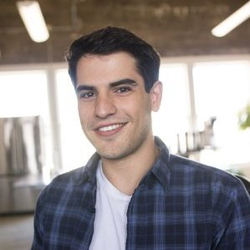# Network Visualization with Saliency Maps, Fooling Images, and Class Visualization

Here we explore the use of image gradients for generating new images. When training a model, we usually define a loss function which measures our current unhappiness with the model’s performance; we then use backpropagation to compute the gradient of the loss with respect to the model parameters, and perform gradient descent on the model parameters to minimize the loss. Here we do something slightly different.

We start from a convolutional neural network model which has been pretrained to perform image classification on the ImageNet dataset. We will use this model to define a loss function which quantifies our current unhappiness with our image, and then use backpropagation to compute the gradient of this loss with respect to the pixels of the image.

We will then keep the model fixed, and perform gradient descent on the image to synthesize a new image which minimizes the loss. We explore three techniques for image generation:

Saliency Maps: Saliency maps are a quick way to tell which part of the image influenced the classification decision made by the network.
Fooling Images: We can perturb an input image so that it appears the same to humans, but will be misclassified by the pretrained network.
Class Visualization: We can synthesize an image to maximize the classification score of a particular class; this can give us some sense of what the network is looking for when it classifies images of that class.

We can observe a few example images from the validation set of the ImageNet ILSVRC 2012 Classification dataset.

Using this pretrained model, we can compute class saliency maps. A saliency map tells us the degree to which each pixel in the image affects the classification score for that image. To compute it, we compute the gradient of the unnormalized score corresponding to the correct class (which is a scalar) with respect to the pixels of the image. If the image has shape (H, W, 3) then this gradient will also have shape (H, W, 3); for each pixel in the image, this gradient tells us the amount by which the classification score will change if the pixel changes by a small amount. To compute the saliency map, we take the absolute value of this gradient, then take the maximum value over the 3 input channels; the final saliency map thus has shape (H, W) and all entries are nonnegative. More information can be found in “Deep Inside Convolutional Networks: Visualising Image Classification Models and Saliency Maps”, ICLR Workshop 2014.

We can visualize some class saliency maps on our example images from the ImageNet validation set.

We can also use image gradients to generate fooling images. Given an image and a target class, we can perform gradient ascent over the image to maximize the target class, stopping when the network classifies the image as the target class. More information can be found in “Intriguing properties of neural networks”, ICLR 2014.

In 9 iterations we can generate a fooling image:

Now on to class visualization. By starting with a random noise image and performing gradient ascent on a target class, we can generate an image that the network will recognize as the target class. Concretely, let $I$ be an image and let $y$ be a target class. Let $s_y(I)$ be the score that a convolutional network assigns to the image $I$ for class $y$; note that these are raw unnormalized scores, not class probabilities. We wish to generate an image $I*$ that achieves a high score for the class $y$ by solving the problem $$I* = \arg\max_I s_y(I) - R(I)$$ where $R$ is a (possibly implicit) regularizer (note the sign of $R(I)$ in the argmax: we want to minimize this regularization term). We can solve this optimization problem using gradient ascent, computing gradients with respect to the generated image. We will use (explicit) L2 regularization of the form $$R(I) = \lambda |I|_2^2$$ and implicit regularization by periodically blurring the generated image. We can solve this problem using gradient ascent on the generated image. More information can bbe found in “Deep Inside Convolutional Networks: Visualising Image Classification Models and Saliency Maps”, ICLR Workshop 2014, or “Understanding Neural Networks Through Deep Visualization”, ICML 2015.

We can observe some images of the training process during the generation of an tarantula image.

We can observe some images of the training process during the generation of a Yorkshire terrier image.##### Eric M. Fischer
###### Ph.D. Statistics with specialization in Artificial Intelligence

My research interests are in natural language processing and generative modeling.